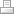## Quantitative Analysis of Unstable Isotope Decay in the Human Body

The human body includes, on average, one gram or more of 15 elements, and less than 1 gram of 44 elements.  Among the top four elements, carbon and hydrogen contain naturally occurring unstable isotopes with half-lives long enough to be statistically present in our bodies, and pose a risk to our DNA composition.

## Carbon Composition

On average, the human body contains 16.5 kilograms (i.e. 32.5 lbs) of carbon.  This would be 8.02x10^26 carbon atoms, and given natural abundance, 8.02x10^14 of these would be unstable Carbon-14 (14C) atoms.  With a half-life of 5.73x10^3 years, there would be 1.40x10^11 14C decays every year, or in smaller scales, 1.60x10^7decays every hour, or 4.44x10^3 every second.

Since the energy of the 14C -> 14N beta particle decay is 0.1564 Million electron Volts (MeV), the total energy released into the body is 2.19x10^10 MeV per year, 2.50x10^6 MeV per hour, or 6.94x10^02 MeV every second.

## Hydrogen Composition

On average, the human body contains 7.0 kilograms (i.e. 15.4 lbs) of hydrogen.  This would be 4.18x10^27 hydrogen atoms, and given natural abundance, 4.18x10^11 of these would be unstable Hydrogen-3 (3H) atoms.  With a half-life of 12.32 years, there would be 3.39x10^10 3H decays every year, 3.87x10^6 decays every hour, or 1.08x10^3 every second.

Since the energy of the 3H -> 3He beta particle decay is 0.01859 MeV, the total energy released into the body is 6.31x10^8 MeV per year, 7.20x10^4 MeV per hour, or 20.0 MeV every second.Print | Sitemap
© 2016-2022 Stable Isotope Foundation LLC -- Not-for-profit technology research & development company dedicated to public benefit.